# Civil Engineering - UPSC Civil Service Exam Questions

36.

The performance of a well is measured by its

 A. Specific capacity B. Specific yield C. Storage coefficient D. Permeability coefficient

Explanation:

No answer description available for this question. Let us discuss.

37.

What is the prime objective of providing a point lock ?

 A. To ensure that each switch is correctly set. B. To ensure that the point may not be operated while the train is on it. C. To detect any obstruction between stock rail and tongue rail. D. To limit the riding of the conical exterior circumference of the wheels.

Explanation:

No answer description available for this question. Let us discuss.

38.

The velocity vector for a steady three-dimensional flow field is described as :
At point (1, 2, 3) what is the approximate value of the magnitude of the velocity?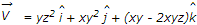A. 21 B. 18 C. 10 D. 4

Explanation:

No answer description available for this question. Let us discuss.

39.

As per the maximum principal stress theory, when a shaft is subjected to a bending moment M and torque T, and if σ is the allowable stress in axial tension, then the diameter 'd' of the shaft is given by

 A.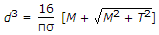B.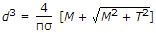C.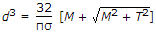D.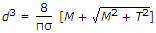Explanation:

No answer description available for this question. Let us discuss.

40.

In a closed traverse, the sum of south latitudes exceeds the sum of north latitudes and the sum of east departures exceeds the sum of west departures. The closing line will lie in the